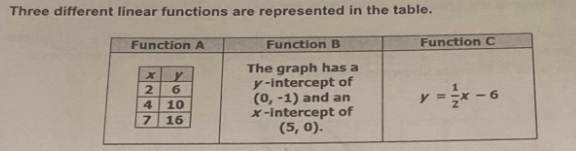### Still have math questions?

Algebra
QuestionThree different linear functions are represented in the table.

Which is the correct order of the functions from least to greatest steepness with respect to the rate of change?

A. Function A, Function B, Function C

B. Function C, Function B, Function A

C. Function B, Function A, Function C

D. Function B, Function C, Function A